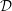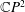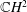Home

### Local differential geometry (53Bxx)

• Article
• ##### Generalized${\mathcal{D}}$ -Einstein Real Hypersurfaces in$\mathbb{C}P^{2}$ and$\mathbb{C}H^{2}$
• Canadian Mathematical Bulletin, Volume 63, Issue 4

### Global differential geometry (53Cxx)

• Article
• ##### SOME$\boldsymbol {L^p}$-HARDY AND$\boldsymbol {L^p}$-RELLICH TYPE INEQUALITIES WITH REMAINDER TERMS
• Journal of the Australian Mathematical Society, First View

• Article
• ##### POWER SERIES PROOFS FOR LOCAL STABILITIES OF KÄHLER AND BALANCED STRUCTURES WITH MILD$\partial \overline {\partial }$-LEMMA
• Nagoya Mathematical Journal, First View

• Article
• ##### REALIZING METRICS OF CURVATURE$\mathbf {\leq -1}$ ON CLOSED SURFACES IN FUCHSIAN ANTI-DE SITTER MANIFOLDS
• Journal of the Australian Mathematical Society, First View

### Symplectic geometry, contact geometry (53Dxx)

• Article
• ##### Pseudorotations of the$2$-disc and Reeb flows on the$3$-sphere
• Ergodic Theory and Dynamical Systems, First View